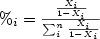## Linear X-Stat Estimator

This is a generalized x-stat estimator that assumes creativename's naive linear model, as opposed to the more complicated and computationally intensive non-linear conditional probability model proposed by King Morgoth. The basis is the assumption that strength ratios are retained.

The formula is:Where X.i are the input values for n inputs, scaled to x-stat values with the strongest in the pack set at 50%.

Enter strength values separated by newline (example values have been pre-filled)
All values are assumed to be percentages. They must be between 0 and 100.
You can also optionally pass names, for example: Link vs. Cloud vs. Snake